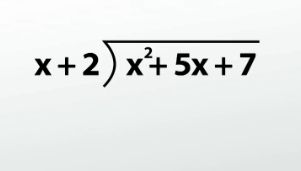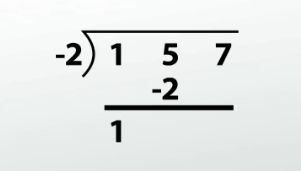# How to Use Synthetic Division to Divide Polynomials

An error occurred trying to load this video.

Try refreshing the page, or contact customer support.

Coming up next: Dividing Polynomials with Long and Synthetic Division: Practice Problems

### You're on a roll. Keep up the good work!

Replay
Your next lesson will play in 10 seconds
• 0:03 Synthetic Division
• 0:24 Synthetically Divide
• 2:42 Missing Parts
• 4:17 Larger Problems
• 6:10 Lesson Summary

Want to watch this again later?

Timeline
Autoplay
Autoplay
Speed

#### Recommended Lessons and Courses for You

Lesson Transcript
Instructor: Kathryn Maloney

Kathryn teaches college math. She holds a master's degree in Learning and Technology.

Synthetic division is a 'short-cut' way of dividing a polynomial by a monomial. You still need to know long division, sorry, but this method is way fun when you're dividing by a monomial!

## Synthetic Division

Many students prefer synthetic division over long division and even argue why they have to learn long division. You need to know long division because synthetic only works when you are dividing by a first degree binomial, for example, (x + 3). If you are dividing by a longer polynomial, say (x2 - 2x + 5), you need to use long division!

## Example #1: How to Synthetically Divide(x2 + 5x + 7) / (x + 2)

At this point, I have seen synthetic division written two ways. The first has the divisor in a half-box on the upper left; the other looks like a division symbol. Both ways are exactly the same, but I prefer the division symbol.

First, if the dividend is not in descending order, we need to do that first. I'll talk about missing parts in the next example. But for now, (x2 + 5x + 7) is in descending order. The divisor also must be in descending order. And it is: (x + 2).

Write your usual long division symbol. Place the coefficients of the dividend under the symbol, just like in long division, but do not write the variables. Make sure to leave space between each number. You don't want to get them confused.

Next, look at x + 2. We're going to write -2 to the left of the long division symbol. You always take the opposite of what you see in the divisor. So inside the long division symbol, we're going to have 1, 5 and 7. On the outside, we're going to have -2.

Our first step is to bring down the leading coefficient, 1.

Multiply 1 * -2, and place the result underneath the next coefficient, 5. So you have -2 * 1, which is -2. 5 + (-2) is 3. (Write that next to the leading coefficient, 1.)Multiply 3 times -2 and place the result underneath the next coefficient, 7. So -2 * 3 is -6. We add straight down and get 1. (So we have 1 written next to the 3.)

In synthetic division, the degree of the final polynomial answer is one less than the dividend polynomial. Since x2 + 5x + 7 is degree 2, our answer will be degree 1. What will this look like?

Starting from the left, we'll have 1x + 3 with a remainder of 1. The remainder will be written the same as if we had done this problem as long division - a fraction.

So our answer is going to look like:

x + 3 + 1 / (x + 2)

## Example #2: Missing Parts

In this example, let's look at a dividend that is missing terms when we write it in descending order.

(x4 + 81) / (x - 3)

These are both written in descending order, but we don't have an x3, x2, or x. In this case, we need to put in a 'place holder' for them. Since we don't have a number, we're going to write 0x3, 0x2, and 0x.

Write the long division symbol. 3 will be our divisor - that's the number that goes in front of the long division symbol. 1, 0, 0, 0, 81 is our dividend. Now, we do synthetic division just like the last one.

We bring down our leading 1.

We multiply 3 * 1, which is 3, and we add down. 0 + 3 = 3, and we do it all over again.

3 * 3 is 9. Add down: 0 + 9 is 9, and do it again.

3 * 9 is 27. 0 + 27 is 27.

To unlock this lesson you must be a Study.com Member.

### Register to view this lesson

Are you a student or a teacher?

### Unlock Your Education

#### See for yourself why 30 million people use Study.com

##### Become a Study.com member and start learning now.
Back
What teachers are saying about Study.com

### Earning College Credit

Did you know… We have over 160 college courses that prepare you to earn credit by exam that is accepted by over 1,500 colleges and universities. You can test out of the first two years of college and save thousands off your degree. Anyone can earn credit-by-exam regardless of age or education level.